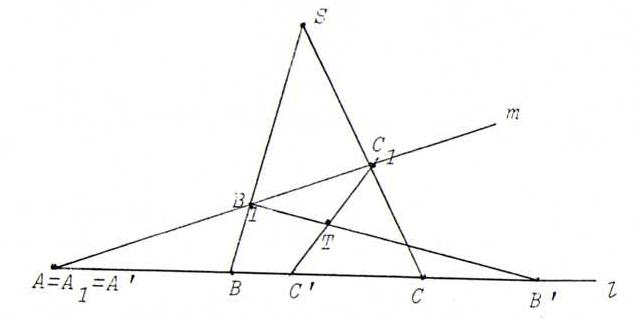### COURSE OF PROJECTIVE GEOMETRY

§ 13:

O52π is the product of the perspectivity ρ from l onto m with center S and the perspectivity σ from m onto l with center T.
So the second fixed point is M := ST.l .

Remark : If we use this with C = B', we find: M = A ↔ (A, B'; B, C') = -1.
Proof:
→ by constructing C' if M = A (so T = B1B'.SA),
← by constructing M if C' is the fourth harmonic with {A, B'} and B (so C' = C1X.l with X = B1B'.SA).

O53
Choose s and m through A and R and S on s as in the picture of §13 such that μ(λ(B)) = B'. Then ψ = μoλ.
Explanation: a parabolic projectivity with fixed point A that maps B to B', maps B' to the fourth harmonic with {A,B'} and B (see the remark after the answer of O52) ; so ψ is unique with these data.

O54
The linear mapping maps b to αc, and c to βb, and a = b - 2c to ρa, so it has matrix ((0, β), (α, 0)) where -2β = ρ , α = -2ρ.
So the matrix is β((0,1),(4,0)).
The eigenvalues are 2 and -2 with eigenspaces E-2 = λ(1,-2) = λa, E2 = λ(1,2) = λ(-2,3,-4). These are also the fixed points.